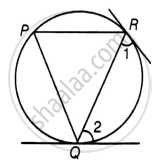# Prove that the tangents drawn at the ends of a chord of a circle make equal angles with the chord. - Mathematics

Sum

Prove that the tangents drawn at the ends of a chord of a circle make equal angles with the chord.

#### Solution

Let QR be a chord in a circle with center O and ∠1 and ∠2 are the angles made by tangent at point R and Q with chord respectively.

To Prove: ∠1 = ∠2Let P be another point on the circle, then, join PQ and PR.

Since, at point Q, there is a tangent.

∠RPQ = ∠2   .....[Angles in alternate segments are equal] [Equation 1]

Since, at point R, there is a tangent.

∠RPQ = ∠1  ......[Angles in alternate segments are equal] [Equation 2]

From Equation 1 and Equation 2

∠1 = ∠2

Hence Proved.

Concept: Concept of Circle - Centre, Radius, Diameter, Arc, Sector, Chord, Segment, Semicircle, Circumference, Interior and Exterior, Concentric Circles
Is there an error in this question or solution?

#### APPEARS IN

NCERT Mathematics Exemplar Class 10
Chapter 9 Circles
Exercise 9.3 | Q 9 | Page 108
Share